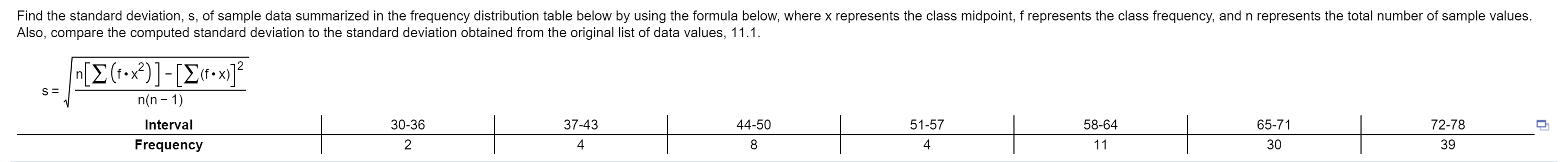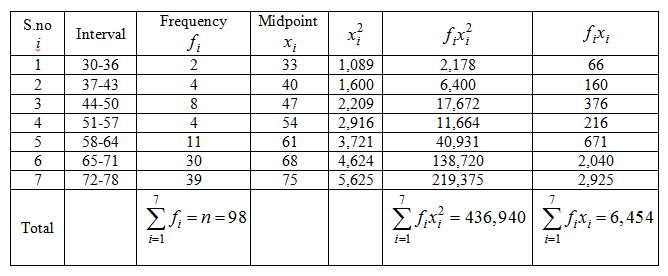# Find the standard deviation, s, of sample data summarized in the frequency distribution table below by using the formula below, where x represents the class midpoint, f represents the class frequency, and n represents the total number of sample values.Also, compare the computed standard deviation to the standard deviation obtained from the original list of data values, 11.1.(f-x)65-713072-7839n(n -158-6451-57444-5030-36237-434IntervalFrequency

Question
3931 viewshelp_outlineImage TranscriptioncloseFind the standard deviation, s, of sample data summarized in the frequency distribution table below by using the formula below, where x represents the class midpoint, f represents the class frequency, and n represents the total number of sample values. Also, compare the computed standard deviation to the standard deviation obtained from the original list of data values, 11.1. (f-x) 65-71 30 72-78 39 n(n -1 58-64 51-57 4 44-50 30-36 2 37-43 4 Interval Frequency fullscreen
check_circle

Step 1

Obtain the calculation table required to find standard deviation for the sample data summarized in frequency distribution.

The data represents the frequencies for the class intervals of sample data.

Consider the midpoints of the class intervals be denoted by xi

The general formula to calculate midpoint is,

xi = (Lower bound + Upper bound)/2.

The frequencies of the class intervals be denoted by fi and the total frequency be denoted by n.

Thecalculation table required to find standard deviation for the sample data summarized in frequency distribution is obtained from the calculation given below:

Step 2

Obtain the standard deviation for the sample data summarized in frequency distribution.

The general formula to obtain standard deviation is,

s = (SQRT((nSfixi^2) – (Sfixi)^2)))/n(n – 1)

The standard deviation for the sample data summarized in frequency distribution is obtained as 11.07519 from the calculation given below:

Step 3

Compare the computed standard deviation to the standard deviation obtained from the original data values.

The standard deviation obtained from the original list of data values is 11.1.

The standard deviation comp...

### Want to see the full answer?

See Solution

#### Want to see this answer and more?

Solutions are written by subject experts who are available 24/7. Questions are typically answered within 1 hour.*

See Solution
*Response times may vary by subject and question.
Tagged in

### Statistics